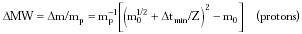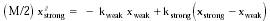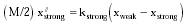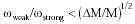Nanomedicine, Volume I: Basic Capabilities

Robert A. Freitas Jr., Nanomedicine, Volume I: Basic Capabilities, Landes Bioscience, Georgetown, TX, 1999

4.4.3 Single-Proton Massometer

A nanopendulum may also be used to measure molecular-scale masses. Torsion, helical spring, and vibrating rod mechanisms all will work, but one of the most efficient designs for weighing the smallest masses may be the coiled suspension-spring cantilever massometer illustrated schematically in Figure 4.6, which is analogous in principle to the traditional quartz crystal microbalance.2896 In this design, a beam of length Lbeam and circular cross-sectional radius Rbeam is hinged at one end and carries a sample holder of solid volume Vsample at the other end. The beam is supported (a variable distance Lspring from the hinge) by a spring consisting of a movable suspension rod of total length lrod, circular cross-sectional radius rrod, and spring constant ks ~ p2 rrod4 E G / (G lrod3 + E p rrod2 lrod) using spring (rod) material of density r, Young's modulus E, and shear modulus G.* The natural resonance period for this unloaded system is T0 = (2 p Lbeam/Lspring) (m0/ks)1/2 = Z m01/2, where Z = (2 Lbeam / Lspring rrod2) ((G lrod3 + E p rrod2 lrod) / E G)1/2 and m0 = r (Vsample + p Lbeam Rbeam2 + p lrod rrod2). Gravitational loading is negligible for suspension system mass m << (ks Lspring2 / g Lbeam2) ~ 10-15 kg; m ~ 10-20 kg in the exemplar system.

* The lrod-3 dependence of ks is not strictly valid for a folded structure because the forces at the end of a folded spring have a smaller mechanical advantage, so the structure acts somewhat stiffer. Further investigation is warranted. However, a large number of alternative low-stiffness structures are available including van der Waals contacts with low loading, gas springs, diamagnetic traps and actively controlled electrostatic traps [J. Soreff, personal communication, 1997].

After adding a test mass Dm to the sample holder, the resonance period increases to Tm = Z (m0 + Dm)1/2, which may be detected if Tm - T0 > Dtmin ~ 1 nanosec, the minimum convenient time interval (Section 10.1). Hence the minimum detectable molecular weight DMW, expressed in proton masses (mp = 1.67 x 10-27 kg), is{Eqn. 4.25}

In Figure 4.6, beam and rod are coiled in two dimensions (although three-dimensional coiling, more difficult to depict, provides maximum compactness). Lbeam and lrod are chosen to minimize total coil area Acoil = 2 Rbeam Lbeam + 2 rrod lrod. Using aluminum for the rod and beam (E = 6.9 x 1010 N/m2, G = 2.6 x 1010 N/m2, r = 2700 kg/m3), Lbeam = 1200 nm, lrod = 1920 nm, rrod = 0.5 nm, Vsample = 1 nm3, and Dtmin = 10-9 sec, then DMW ~ 1 proton for Lspring = 1 nm >> Dxmin = 0.01 nm, the minimum measurable displacement (Section 4.3.1). (For Lspring > 1 nm, physiological ~1 g accelerative loads on a ~1 micron nanorobot in which the sensor is embedded produce undetectable accelerative beam displacements < Dxmin.)

Moving the suspension rod closer to the sample holder reduces device sensitivity. For Lspring = Lbeam, minimum DMW ~ 1200 protons, permitting moderately large molecules to be weighed in minimum time (~10-6 sec). Using a very thin sensor configuration (hsensor~20 nm) and coils occupying ~50% of sensor cavity space, sensor dimensions are (79 nm)2 x 20 nm giving a massometer volume ~105 nm3 or ~0.01% of the total volume of a 1 micron3 nanorobot. Sensor mass is ~ 3 x 10-19 kg, including diamondoid housing and prorated share of support mechanisms. If one measurement cycle including sample loading/unloading operations can be completed every ~10 microsec, then the device processes its own mass of glucose molecules in ~10 sec. Note that biological molecules weighed in air or in vacuo may lose water of hydration, whereas weighing in water presents other complications that merit further investigation.

J. Soreff points out that for a molecule on a spring, the precision of the measurement is linked to the sharpness of the resonance, which is optimized to the degree the vibrational frequency of the spring/molecular mass system is kept well below the vibrational resonance frequencies of the molecule itself. The following discussion reports the results of a simple lumped-element analysis of the effects of internal vibration on the massometer completed for the author by J. Soreff, which confirms the previously described performance estimates for the massometer.

Suppose that it is desired to weigh some mass M to a precision DM by attaching it to a weak measuring spring (spring constant kweak) and measuring the shift in resonant frequency. The shift in frequency due to coupling to an internal mode of the sample must be less than (1/2) (DM / M) (1 / 2 p) (kweak/M)1/2. If the sample has some lowest internal mode frequency nint, then approximating the sample as two M/2 masses separated by xstrong connected by a strong spring (kstrong) gives nint = (1/p) (kstrong/M)1/2. If this sample is attached to a weak measuring spring of length xweak, which is in turn attached to a rigid support, then the equations of motion are:{Eqn. 4.26}{Eqn. 4.27}

Setting xweak = est and xstrong = R est where t = time, s = time constant, and R = -(1 + (2 kweak / M s2)), and since the angular frequencies of the two springs are wstrong = 2 p nint = 2 (kstrong/M)1/2 and wweak = (kweak/M)1/2, then the lower frequency solution for wweak << wstrong may be approximated by s = i wweak (1 - wweak2 / 2 wstrong2) and so the (undesired) fractional change in frequency due to the flexibility of the object to be weighed is{Eqn. 4.28}

For a molecule sample size of Lsample, nint ~ vsound / Lsample ~ 500 GHz for vsound ~ 1500 m/sec and Lsample ~ 3 nm; M = r Lsample3 = 2.7 x 10-23 kg for r = 1000 kg/m3. To detect the mass difference of a single proton in a sample molecule, sensor Q = M / mp = 16,000; from Eqn. 4.28, the sensor may operate at a factor of Q1/2 below nint, or nspring ~ nint / Q1/2 ~ 4 GHz. A period of 0.25 nanosec is slightly too short to allow a phase shift of Dtmin ~ 1 nanosec, since the period is only one-quarter of this. However, a phase shift of p radians should accumulate in tmeas = Q / 2 nspring ~ 2 microsec and should be detectable by looking at magnitudes of forces. Interestingly, an SPM microprobe of circular cross-section and exponential profile with a 0.1 nm2 tip has been proposed that could also reach ~proton mass detection sensitivity at room temperature and 1 atm pressure with an eigenmode frequency of 10 GHz;1195 an electrically-driven carbon nanotube-based resonant-beam balance demonstrated ~10-17 kg (~1010 proton or ~0.01 micron3 mass) sensitivity in 1999.3023

Existing surface acoustic wave (SAW) devices458 are chemical sensors that can measure areal mass densities as small as 80 picogram/cm2, or 0.01 N2 molecule per nm2 of surface, though current devices are limited to ~micron2 areas. A proposed quartz microresonator microbalance could detect a 10-6 molecular monolayer,459 the equivalent of 5 x 10-9 molecule per nm2. Detection of ~400 million protons using an MRFM vibrating rod ~60 nm thick was demonstrated by Rugar3256 in 1998.

Last updated on 17 February 2003